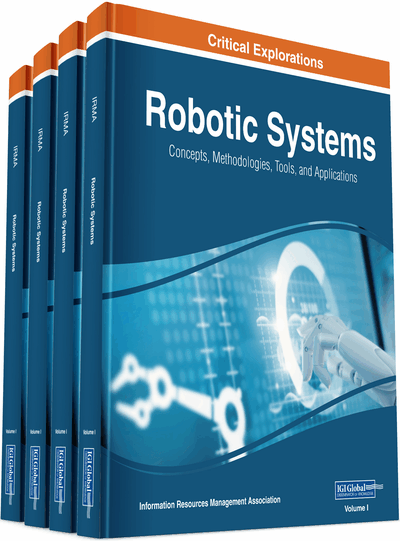# Modelling, Control and Prediction using Hierarchical Fuzzy Logic Systems: Design and Development

Masoud Mohammadian (University of Canberra, Australia)
DOI: 10.4018/978-1-7998-1754-3.ch009
Available
\$29.50
No Current Special Offers

## Abstract

Hierarchical fuzzy logic systems are increasingly applied to solve complex problems. There is a need for a structured and methodological approach for the design and development of hierarchical fuzzy logic systems. In this paper a review of a method developed by the author for design and development of hierarchical fuzzy logic systems is considered. The proposed method is based on the integration of genetic algorithms and fuzzy logic to provide an integrated knowledge base for modelling, control and prediction. Issues related to the design and construction of hierarchical fuzzy logic systems using several applications are considered and methods for the decomposition of complex systems into hierarchical fuzzy logic systems are proposed. Decomposition and conversion of systems into hierarchical fuzzy logic systems reduces the number of fuzzy rules and improves the learning speed for such systems. Application areas considered are: the prediction of interest rate and hierarchical robotic control. The aim of this manuscript is to review and highlight the research work completed in the area of hierarchical fuzzy logic system by the author. The paper can benefit researchers interested in the application of hierarchical fuzzy logic systems in modelling, control and prediction.
Chapter Preview
Top

## Introduction

The problem of controlling uncertain dynamic systems which are subject to external disturbances, uncertainty and sheer complexity is of considerable interest. Conventional modelling approaches employ mathematical models and examine the system’s evolution and its control. Such approaches are not completely successful when applied to large non-linear complex systems. These models work well provided the system meet the requirement and assumption of synthesis techniques. However due to uncertainty and sheer complexity of the actual dynamic system, it is very difficult to ensure that the mathematical model does not break down (Mohammadian & Stonier, 1995).

Progress in solving these problems has been with the aid of new advanced high-speed computers and the application of artificial intelligence paradigms, particularly neural networks, fuzzy logic systems and evolutionary algorithms. Fuzzy logic systems have been successfully applied in the place of the complex mathematical systems and they have numerous practical applications in modelling, control, prediction and automation. They have been found useful when the system is either difficult to predict and or difficult to model by conventional methods.

Fuzzy set theory (Zadeh, 1965) provides a mean for representing uncertainties. The underlying power of fuzzy logic is its ability to represent imprecise values in an understandable form. Fuzzy logic systems have a fuzzy knowledge base (FKB) consisting of a set of fuzzy rules of the form:

whereare normalised fuzzy sets for n input variables xk, k=1,…,n, and whereare normalised fuzzy sets for m output variables zk, k=1,…,m. The inference engine of fuzzy logic system applies the fuzzy rules to output action/s from inputs. There are many types of inference engines in the literature, including the popular Mamdani inference engine.

Given a fuzzy knowledge base with M rules and n antecedent variables, a fuzzy logic system as given in Equation 1 uses a singleton fuzzifier, Mamdani product inference engine and centre average defuzzifier to determine output variables, has the general form for a single output variable, say zk:

(1) whereare centres of the output setsand membership function μ defines for each fuzzy setthe value of xi in the fuzzy set, namely,.

Common shapes of the membership function are triangular, trapezoidal and Gaussian membership functions.

## Complete Chapter List

Search this Book:
Reset